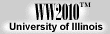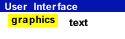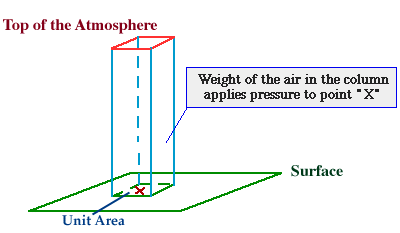.
Atmospheric Pressure
force exerted by the weight of the air

Atmospheric pressure is defined as the force per unit area exerted against a surface by the weight of the air molecules above that surface. In the diagram below, the pressure at point "X" increases as the weight of the air above it increases. The same can be said about decreasing pressure, where the pressure at point "X" decreases if the weight of the air above it also decreases.Think in terms of air molecules...if the number of air molecules above a surface increases, there will be more molecules to exert a force on that surface and the pressure increases. The opposite is also true, where a reduction in the number of air molecules above a surface will result in a decrease in pressure. Atmospheric pressure is measured with an instrument called a barometer, which is why atmospheric pressure is also referred to as barometric pressure.In aviation and television weather reports, pressure is referred to in inches of mercury ("Hg), while meteorologists use millibars (mb), the unit of pressure found on weather maps.

As an example, let us consider a "unit area" to be 1 square inch. At sea level, on average, the weight of the air above this unit area would weigh 14.7 pounds! The pressure applied by this air on the unit area would be 14.7 pounds per square inch. Meteorologists use a metric unit for pressure called a millibar. Average pressure at sea level is 1013.25 millibars.

 Terms for using data resources. CD-ROM available. Credits and Acknowledgments for WW2010. Department of Atmospheric Sciences (DAS) atthe University of Illinois at Urbana-Champaign.